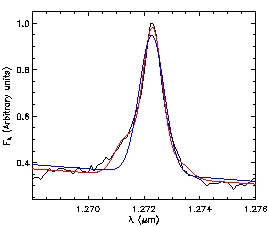HOME RESEARCH CV ENSINO     (TEACHING) SOFTWARE LINKS
Emission-line PROfile FITting routine - PROFITThe PROFIT is an IDL routine to do automated fitting of emission-line profiles by Gaussian curves or Gauss-Hermite series otimized for use in Integral Field and Fabry-Perot data cubes. As output PROFIT gives two-dimensional fits files for the emission-line flux distribution, centroid velocity, velocity dispersion and higher order Gauss-Hermite moments (h3 and h4).

To run PROFIT the following routines are required:

• MINPACK (Moré, Garbow & Hillstrom, 1980) Levenberg-Marquardt nonlinear least-squares optimization algorithm. The mpfitfun.pro routine can be found here .

• The IDL Astronomy User's Library, which can be downloaded from here.

If you used the PROFIT routine I would appreciate if you cite the following paper: Riffel (2010, Ap&SS, 327, 239)